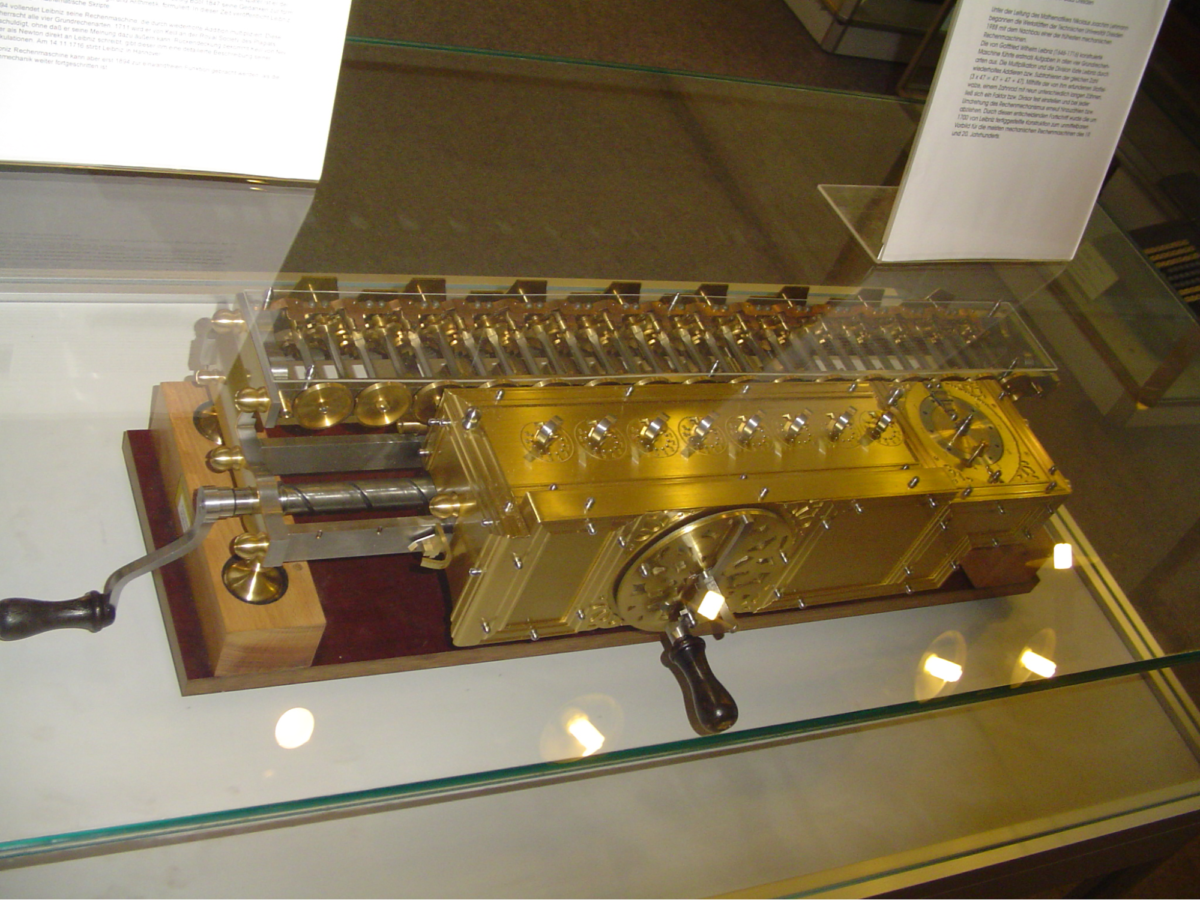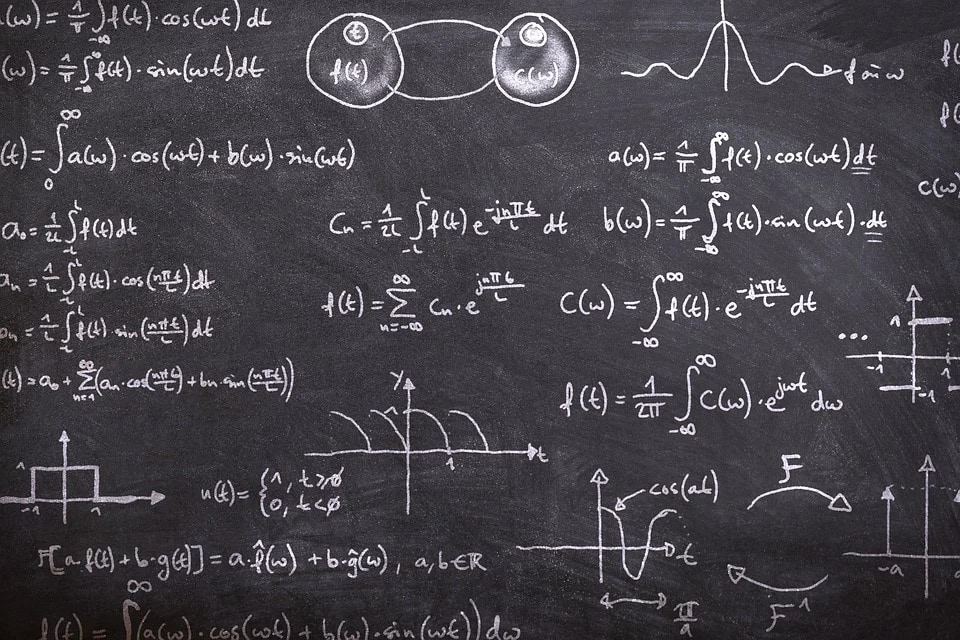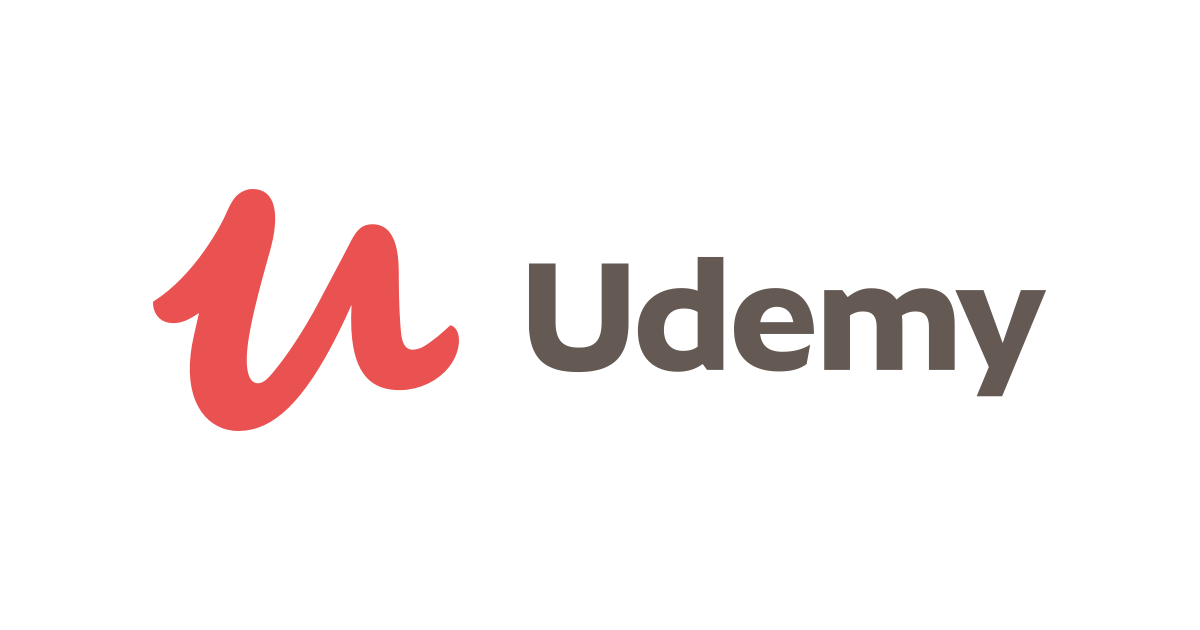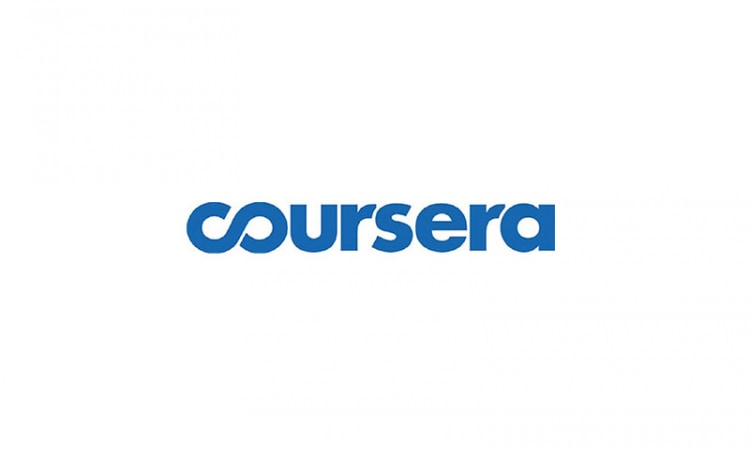Thursday, March 30, 2023
HomeAIExplain mathematics for machine learning | Recommended books and courses are also...

# Explain mathematics for machine learning | Recommended books and courses are also introduced!

In this article, I will explain how to face mathematics when studying machine learning.

I think that for beginners, especially those who do not specialize in science subjects such as humanities, there is always an image that mathematics is difficult and difficult to get into.

In this article, I will introduce the mathematics roadmap and study methods necessary for machine learning. I would appreciate it if you could help me study even a little.

• The relationship between machine learning and mathematics
• Why You Should Learn Mathematics in Machine Learning
• History of Computers and Mathematics
•  History of Machine Learning and Mathematics
• Points for learning mathematics in machine learning
• Does Machine Learning Need Mathematics?
• Knowledge of mathematics is essential for a deeper understanding of machine learning results
• Deep Learning Requires More Complex Mathematics
• What kind of mathematics is needed for machine learning?
• Content up to junior high and high school mathematics
• Linear algebra / Probability/Statistics / Analysis (calculus)
• Other/developmental content
• How to study mathematics in machine learning
• learn from books
• learn in an online course
• study online at university
• in conclusion

## The relationship between machine learning and mathematics

Machine learning and mathematics are inextricably linked. This section introduces the relationship between machine learning and mathematics.

### Why You Should Learn Mathematics in Machine Learning

Knowledge of mathematics is essential to understanding machine learning. Machine learning ideas often derive from mathematical discoveries, and mathematics and computer science have developed while mutually influencing each other.

However, just because knowledge of mathematics is necessary to understand machine learning does not mean that “all people who deal with machine learning must study mathematics”. We will discuss this point in more detail in the next section.

### History of Computers and Mathematics

Let’s look at the close relationship between machine learning and mathematics from a historical perspective.Source: https://commons.wikimedia.org/wiki/File:Boulier1.JPG

If you translate computer into Japanese, it will be a calculator. The idea of ​​a calculator itself has been around for a long time, and calculators like the Japanese abacus are scattered all over the world.Source: https://ja.wikipedia.org/wiki/%E3%83%95%E3%82%A1%E3%82%A4%E3%83%AB:Leibnitzrechenmaschine.jpg

The idea of ​​the computer as a mechanical calculator was conceived by 17th-century mathematicians Blaise Pascal and Godfried Leibniz. By the way, Leibniz is also the creator of the binary system.

These calculators are not yet programmed, but they can do simple calculations automatically.

#### Discovery of “Computability”

Computers, like the ones we use every day, were invented in the early 20th century. Mathematician Turing devised an abstract machine called a Turing machine, in which hardware and software perform operations according to a program.

Turing machines were invented to explain the concept of computability. This rigorously defined the computation.

### History of Machine Learning and MathematicsMathematician von Neumann realized a computer as a machine consisting of a large number of vacuum tubes and cables based on the idea of ​​a Turing machine. This computer is called a Von Neumann computer, and most modern computers use the Von Neumann computer method.

#### checker programNeumann gave computers enormous computational power. Arthur Samuel came up with the idea of ​​machine learning in response to the ever-increasing computational power of computers.

He developed a checkers program that solves chess problems, and is said to be the world’s first learning program. This era corresponds to the first AI boom in the history of AI. In response to this first AI boom, machine learning research has become active, and has reached the present after several breakthroughs.

## Points for learning mathematics in machine learningBefore moving on to specific areas of mathematics, I would like to introduce some points to consider when studying mathematics for machine learning.

### Does Machine Learning Need Mathematics?

In the first place, it is possible that you do not need to study mathematics.

Most machine learning libraries are designed in such a way that they can be run without any knowledge of mathematics. So for those who want to use machine learning as a tool to compute simple classifications and inferences, studying mathematics can be a worthless endeavor.

Of course, there is nothing wrong with studying, but the template function of the machine learning library is so good that some people may not need to force themselves to learn mathematics.

#### AI technology utilization service that does not require mathematics or programmingFor example, MatrixFlow Inc. is developing a platform for utilizing AI without specialized knowledge of mathematics, programming, or machine learning.

As machine learning libraries continue to develop, users will be able to do many things without any knowledge of mathematics.

### Knowledge of mathematics is essential for a deeper understanding of machine learning results

Earlier, I said, “Some people don’t need to force themselves to learn mathematics.” Math knowledge is essential when thinking about problems such as “What should I do?”

In fact, you won’t get much out of simple machine learning. You can reap the full benefits of machine learning by digging deeper into your calculations through trial and error over and over again.

### Deep Learning Requires More Complex Mathematics

The emergence of deep learning technology triggered the third AI boom. Deep learning can handle much larger data sets than traditional machine learning, while doing more complex calculations internally.

Deep learning is an advanced technology, and it can be said that it is preferable to have knowledge of mathematics in order to handle it. If you’re working with deep learning in addition to basic machine learning, I’d recommend working on more advanced mathematics studies.

## What kind of mathematics is needed for machine learning?

In this chapter, we will list the mathematics areas that should be studied in machine learning at once.

Some readers may wonder, “Do I have to study all this?” If you try to study everything at once, it will be difficult and exhausting, so it is important to identify the areas that you really need and study.

Now, I will briefly explain the fields of mathematics necessary for learning machine learning.

### Content up to junior high and high school mathematics

In order to understand mathematics in machine learning, knowledge of mathematics learned in junior high school and high school is essential.

By firmly understanding middle school and high school mathematics, you can partially understand how machine learning works and what ideas it is based on.

Once you have solidified your knowledge of junior high and high school mathematics, let’s move on to university mathematics. The field of mathematics at university is roughly divided into three basics: linear algebra, probability/statistics, and analysis (calculus).

### Linear algebra / Probability/Statistics / Analysis (calculus)

In general science universities, first and second year students learn linear algebra and calculus as general education subjects.

#### linear algebra

In high school, we mainly learn geometric vectors, but in college mathematics, we deal with more abstract linear spaces and vector spaces and learn various operations on them.

The fields of machine learning and linear algebra are closely related. Knowledge of matrix calculus is essential to understanding how computers work with data and how they optimize parameters in machine learning.

#### Analysis (calculus)

Differentiation is the science that deals with local changes, and integral is the science that deals with the accumulated amount of those changes. In high school mathematics, differential and integral calculations were performed under limited conditions with few variables, but machine learning deals with more complex calculations.

#### Probability/Statistics

To understand machine learning, knowledge of probability and statistics is essential. The technical goal of machine learning is to learn large amounts of data to reduce the error between predicted and actual values. By studying probability and statistics, you can understand the process theoretically.

### Other/developmental content

Although the content is not limited to machine learning, we will introduce mathematics fields that are generally considered necessary for understanding the IT field and programming.

The field of “algorithms and data structures” is the study of mathematical explanations of the internal processes of computers. Especially for those who are doing competitive programming, it may be a familiar field. Mathematics that generalizes this a little more is “discrete mathematics” and “graph theory”.

In the previous section, I introduced that computer theory can be traced back to set theory and logic.

In order to understand this area, knowledge of “logic circuits”, “formal languages ​​and automata”, and “algebra” is required. Those who want a fundamental understanding of computers should study these areas.

## How to study mathematics in machine learning

Now, I will introduce the recommended study method of mathematics in machine learning. It is good to find a study method that suits you and study intensively, or it is good to study mathematics from various angles with various approaches.

### learn from books

The first method is to learn from books.

The book is excellent in accuracy and richness of information. You can also choose the difficulty of the book according to your knowledge. Many of the books for beginners explain difficult definitions and mathematical formulas in an easy-to-understand manner using diagrams and illustrations, while the books for advanced users carefully explain even the details, giving a deeper understanding of the essence of mathematics. It is a content that approaches.

The following six books are highly recommended.

#### Fermat’s Last Theorem

In order to study mathematics, it is more important than anything else to love studying mathematics. So, let me introduce you to Simon Singh’s Fermat’s Last Theorem, a book that will help you fall in love with studying mathematics.

This book is a non-fiction work that depicts the drama of mathematicians surrounding Fermat’s Last Theorem, which is said to be the biggest unsolved problem in mathematics.

It’s a fascinating work that stimulates interest in mathematics, so it’s a recommended book for those who are about to start studying mathematics in earnest.

#### Mathematics book for artificial intelligence programming

As the cover says, “You can learn the mathematics of artificial intelligence from scratch with this one book!”

In the second half of the book, python code is written, and by running it, you can also study the actual artificial intelligence machine learning process. This is a standard book that covers a wide range of topics from basics to applications.

#### Deep Learning from Scratch ―Theory and Implementation of Deep Learning in Python

This book provides a simple explanation of the mathematics required for machine learning and the more complex deep learning. You can learn what formulas are used in machine learning and deep learning processes while actually writing code.

The above two books are books that focus on the knowledge of mathematics necessary for machine learning, but it is possible to acquire deeper knowledge by studying mathematics professionally with the following three books as a trigger.

#### chart formula series university liberal arts linear algebra

This is a linear algebra reference book published in 2020 by the chart series, a standard reference book for high school mathematics. This is a reference book that focuses on practicing problems specific to chart expressions, and by studying this book step by step, you will steadily acquire knowledge of linear algebra.

#### chart formula series college education calculus

This is the calculus of the blue chart. The introductory part carefully bridges high school mathematics and university mathematics. There are plenty of problems and detailed explanations, so you can use it as a problem collection for doing a lot of calculations.

#### Probability statistics for programming

Knowledge of probabilistic statistics is indispensable not only for machine learning, but also for problems that people called “programmers” tackle. Although the content is not easy, you can deepen your knowledge of probability statistics with detailed explanations and supplementary explanations from a programming perspective.

This is a good book that not only covers the basics but also tries to get to the essence of the definition of probability statistics.

### learn in an online course

The second method is to study online.

It is recommended for those who want to set a fixed pace and study steadily, and for those who think that reading a book all at once is a high hurdle. Online courses are not only free content, but also have a wealth of paid content that comes with services such as classes by famous instructors and personal guidance.

Here are three of our favorite online courses:

#### Math Course for AI (Udemy)Udemy is an online learning platform used by 50 million people worldwide with over 150,000 published courses.

One of the most recommended Udemy courses is “Mathematics Course for AI (paid)”. The course does not presuppose programming knowledge, and provides detailed explanations starting from junior high school mathematics knowledge, and the mathematics knowledge necessary for studying AI is explained in an easy-to-understand manner through videos.

In addition, Udemy has a lot of courses related to machine learning, so if you are interested, you should check it out.

#### Chainer Tutorial

Among the free online courses, the “ Chainer Tutorial” is particularly recommended.

The Chainer tutorial provides a general overview of the mathematics knowledge required for machine learning.

The strength of the Chainer tutorial is that you can implement what you learned in the math course in the subsequent sections while studying the python library.

Another great attraction of Chainer tutorials is that you can acquire practical skills in data analysis and deep learning while studying mathematics.

#### KIKAGAKU

Kikagaku is a service famous for AI and machine learning online courses. This course is not a mathematics-specific course, but a professional instructor explains the general knowledge of machine learning, including mathematics, in an easy-to-understand manner.

A feature of Kikagaku is that many companies participate as clients and partners, and it is used for human resource development by many companies.

The third is to learn from youtube videos.

Recently, experts in various fields have commented on youtube channels and put up a lot of videos. It may not be long before youtube becomes a standard learning tool.

#### [Understand in 9 minutes] Thorough explanation of the basics of mathematics necessary for studying AI and machine learning! Calculus, Linear Algebra, Probability Statistics

This video explains the mathematics knowledge necessary for studying machine learning in an easy-to-understand manner by dividing it into three parts: calculus, linear algebra, and probability statistics. I recommend it because it is short and to the point.

#### Introduction to mathematics for AI (artificial intelligence) starting from junior high school mathematics

This video explains the knowledge of mathematics necessary especially when programming AI. It is easy to understand and carefully explained from junior high school mathematics, so it is especially recommended for those who are not good at mathematics.

In addition, recommended reference books are introduced on the homepage of Yobinori, the channel that posted this video . You may want to refer to this along with other videos.

### study online at university

Some university courses are free and open to the public. It covers a wide range of topics, from basic to highly advanced.

Here are three of the most popular online college courses .

#### University of Tsukuba Open CoursewareThe University of Tsukuba Open Courseware is a service that makes the content of classes held at the University of Tsukuba available to the general public.

A particularly recommended course is Professor Jun Sakuma’s machine learning course. This course explains the advanced mathematics necessary for understanding machine learning. It is recommended because it gives you free access to lectures that are actually being held at the university.

#### Deep Learning Basic Course Exercise Contents

Learning content developed by the Matsuo Laboratory at the University of Tokyo. We specialize in deep learning within machine learning.

#### Coursera machine learning courseMachine learning courses of many overseas universities are published on coursera. One of the most famous is the lecture by Andrew Ng, Lecturer at Stanford University. From basics to applications of machine learning, you will receive in-depth lectures from both mathematics and programming perspectives.

## in conclusion

So far, I have introduced how to study mathematics in machine learning. Understanding machine learning requires a fairly extensive knowledge of mathematics, and is often not straightforward.

RELATED ARTICLES